Courses

# Doc: Crystal Lattice and Unit cells Class 12 Notes | EduRev

## Class 12 : Doc: Crystal Lattice and Unit cells Class 12 Notes | EduRev

The document Doc: Crystal Lattice and Unit cells Class 12 Notes | EduRev is a part of the Class 12 Course Chemistry for JEE.
All you need of Class 12 at this link: Class 12

What is a Crystal?

Crystalline solid consists of a large number of small units, called crystals, each of which possesses a definite geometric shape bounded by plane faces. The crystals of a given substance produced under a definite set of conditions are always of the same shape.

Characteristics of Crystal Lattice

• In a crystal lattice, each atom, molecule or ions (constituent particle) is represented by a single point.
• These points are called lattice site or lattice point.
• Lattice sites or points are together joined by a straight line in a crystal lattice.
• When we connect these straight lines we can get a three-dimensional view of the structure. This 3D arrangement is called Crystal Lattice also known as Bravais Lattices.

What is a Unit Cell?

This smallest amount of the solid whose properties resemble the properties of the entire solid irrespective of the amount taken is called a unit cell.

• It is the smallest repeating unit of the solid. Any amount of the solid can be constructed by simply putting as many unit cells as required.
• We would be studying certain properties of a solid which depend only on the constituents of the solid and the pattern of arrangement of these constituents.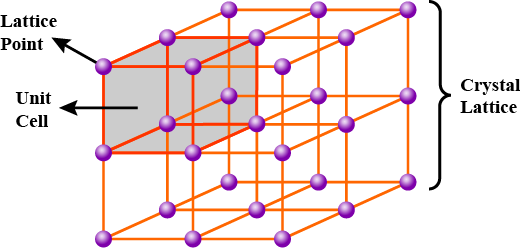A unit cell is the shortest portion of a lattice
• To study these properties, it would be convenient to take up a small amount of the solid as possible because this would ensure that we deal with only the minimum number of atoms or ions.

Characteristics of Unit Cell

The following characteristics define a unit cell:

• A unit cell has three edges a, b, and c, and three angles α, β, and γ between the respective edges.
• The a, b and c may or may not be mutually perpendicular.
• The angle between edge b and c is α, a and c is β and of between a and b is γ.

Types of Unit Cell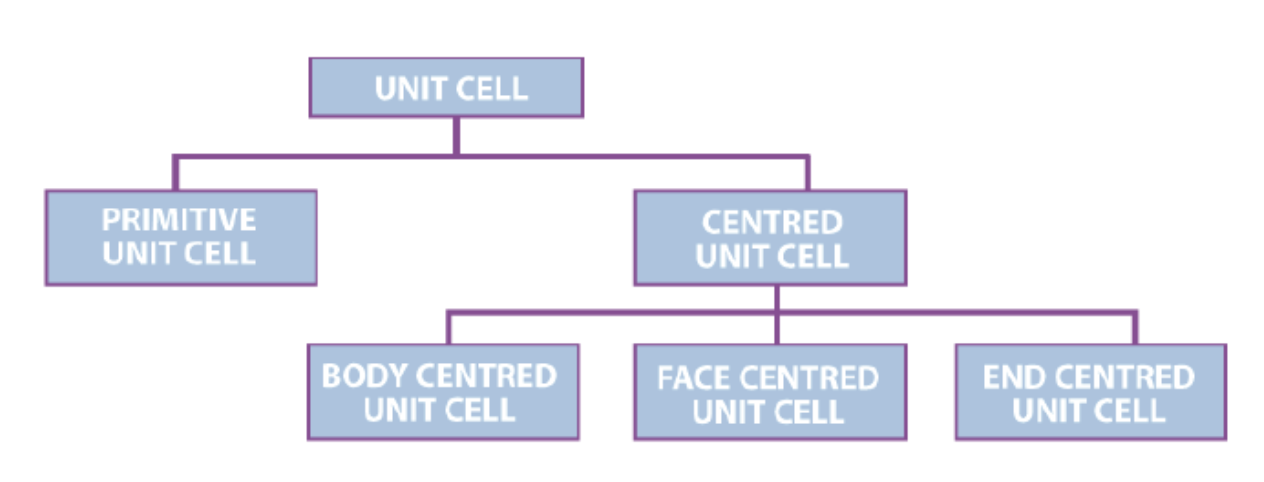1. Primitive Unit Cells

When the constituent particles occupy only the corner positions, it is known as Primitive Unit Cells.

2. Centred Unit Cells

When the constituent particles occupy other positions in addition to those at corners, it is known as Centred Unit Cell.

There are 3 types of Centred Unit Cells:

(i) Body Centred: When the constituent particle at the centre of the body, it is known as Body Centred Unit cell.

(ii) Face Centred: When the constituent particle present at the centre of each face, it is known as the Face Centred Unit cell.

(iii) End Centred: When the constituent particle present at the centre of two opposite faces, it is known as an End Centred Unit cell.

Number of Atoms & Packing Fraction in a Unit Cell
1. Simple Cubic (SC) Unit Cell

• A simple cubic unit cell consists of eight corner atoms. In a simple cubic unit cell, a corner atom touches with another corner atom.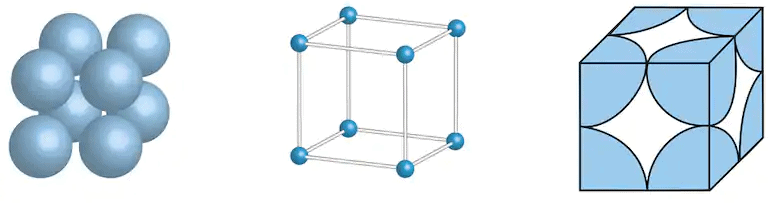Simple cubic unit cell of a crystal lattice
• Number of Atoms in a Simple Cubic Unit Cell
A simple cubic unit cell consists of 8 corner atoms. Each and every corner atom is shared by eight adjacent unit cells. Therefore, one corner atom contributes 1/8th of its parts to one unit cell. Since there are eight corner atoms in a unit cell, the total number of atoms is (1/8)* 8=1. Therefore, the number of atoms in a simple cubic unit cell is one.
In a simple cubic lattice, a corner atom touches with another corner atom.
Therefore, 2r=a. So, the atomic radius of an atom in a simple cubic unit cell is a/2.
• Coordination Number
Consider a corner atom in a simple cubic unit cell. It has four nearest neighbours in its own plane. In a lower plane, it has one more nearest neighbour and in an upper plane, it has one more nearest neighbour. Therefore, the total number of the nearest neighbour is six.
• Packing Density
The packing density of a simple cubic unit cell is calculated as follows:
The unit cell Number of atoms
p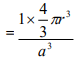Substituting, r=a/2, we get,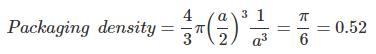The packing density of a simple cubic unit cell is 0.52. It means, 52% of the volume of the unit cell is occupied by atoms and the remaining 48% volume is vacant.

Try yourself:The volume occupied by atoms in a simple cubic unit cell is (edge length = a):

2. Body-Centred Cubic Unit Cell

• A Body-Centered unit cell is a unit cell in which the same atoms are present at all the corners and also at the centre of the unit cell and are not present anywhere else.
• This unit cell is created by placing four atoms that are not touching each other.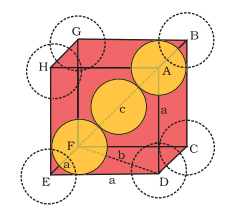Body-centred cubic unit cell

• Then we place an atom on top of these four. Again, four spheres eclipsing the first layer are placed on top of this.
• The effective number of atoms in a Body-Centered Cubic Unit Cell is 2 (One from all the corners and one at the centre of the unit cell).
• Moreover, since in BCC the body-centred atom touches the top four and the bottom four atoms, the length of the body diagonal (√3a) is equal to 4r.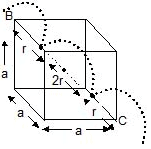The packing fraction in this case is  =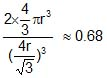VF ≈ 0.32

Try yourself:In a body centered cubic cell, an atom at the body center is shared by:

3. Face Centered Cubic (FCC) Unit Cell

• In an fcc unit cell, the same atoms are present at all the corners of the cube and are also present at the centre of each square face and are not present anywhere else.
• The effective number of atoms in fcc is 4 (one from all the corners, 3 from all the face centres since each face-centred atom is shared by two cubes).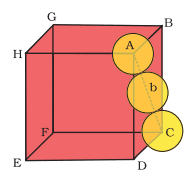Face centered cubic unit cell

• Since, here each face-centred atom touches the four corner atoms, the face diagonal of the cube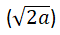is equal to 4r.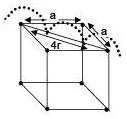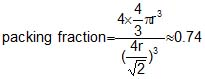.
VF ≈ 0.26

Try yourself:The coordination number of an atom in a fcc lattice is _________.

4. End Centered Cubic (FCC) Unit Cell

A unit cell with a single constituent particle of atom, molecules, or ions located in the centre of the opposite faces, apart from that present in the corners. Two atoms are present in end centred cubic unit cell.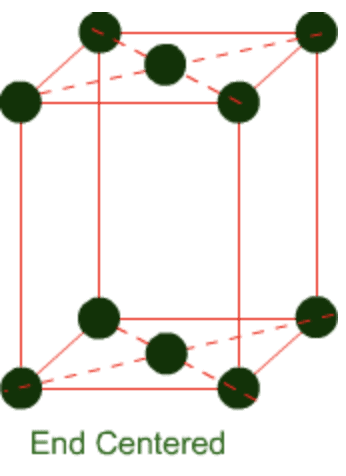5. Hexagonal Primitive Unit Cell

Each corner atom would be common to 6 other unit cells, therefore their contribution to one unit cell would be 1/6. Therefore, the total number of atoms present per unit cell effectively is 6.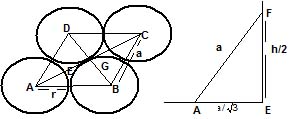In the figure, ABCD is the base of the hexagonal unit cell AD=AB=a. The sphere in the next layer has its centre F vertically above E and it touches the three spheres whose centres are A, B, and D.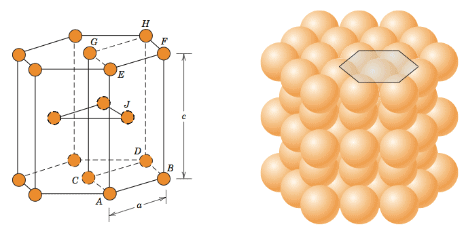Hexagonal close-packing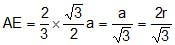Hence,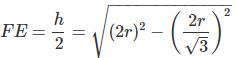The height of the unit cell (h) =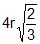The area of the base is equal to the area of six equilateral triangles, =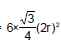.
The volume of the unit cell =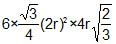.
Therefore,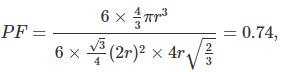VF ≈ 0.26

The Density of Crystal Lattice

The density of crystal lattice is the same as the density of the unit cell which is calculated as: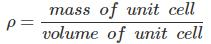=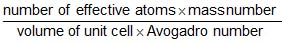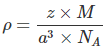Here,
Z = no. of atoms present in one unit cell
m = mass of a single atom
Mass of an atom present in the unit cell = m/NA

Bravais Lattices

Bravais (1848) showed from geometrical considerations that there are only seven shapes in which unit cells can exist.

These are

• Cubic
• Orthorhombic
• Rhombohedral
• Hexagonal
• Tetragonal
• Monoclinic
• Triclinic

Moreover, he also showed that there are basically four types of unit cells depending on the manner in which they are arranged in a given shape. These are:

• Primitive
• Body-Centred
• Face Centered
• End Centered

He also went on to postulate that out of the possible twenty-eight unit cells (i.e. seven shapes, four types in each shape = 28 possible unit cells), only fourteen actually would exist. These he postulated based only on symmetry considerations. These fourteen unit cells that actually exist are called Bravais Lattices.

Seven Crystal Systems

The seven crystal systems are given below:

A. Cubic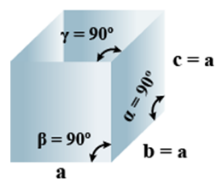• Bravais Lattices
Primitive, face Centered, Body Centered = 3
• Parameters of Unit Cell
(i) Intercepts: a=b=c
(ii) Crystal angle: α = β = γ = 90o
Example: Pb, Hg, Ag, Au, Diamond, NaCl, ZnS

B. Orthorhombic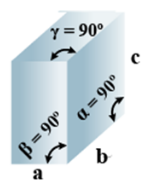• Bravais Lattices:
Primitive, Face Centered, Body-Centered, End Centered = 4
• Parameters of Unit Cell:
(i) Intercepts: a ≠ b≠ c
(ii) Crystal angle: α = β = γ = 90o
Example: KNO2, K2SO4

C. Tetragonal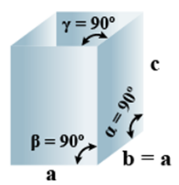• Bravais Lattices:
Primitive, Body-Centered =2
• Parameters of Unit Cell:
(i) Intercepts: a = b≠ c
(ii) Crystal angle: α = β = γ = 90o
Example: TiO2, SnO2

D. Monoclinic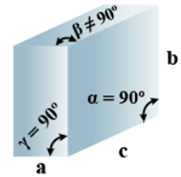• Bravais Lattices
Primitive, End Centered =2
• Parameters of Unit Cell
(i) Intercepts: a ≠ b≠ c
(ii) Crystal angle: α =  γ = 90o
Example: CaSO4.2H2O

E. Triclinic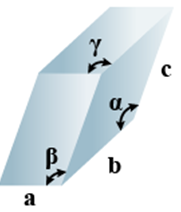• Bravais Lattices
Primitive = 1
• Parameters of Unit Cell
(i) Intercepts: a ≠ b≠ c
(ii) Crystal angle:  α  ≠ β ≠ γ
Example: K2Cr2O7, CaSO5H2O

F. Hexagonal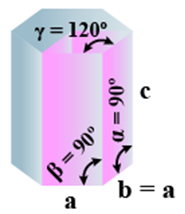• Bravais Lattices
Primitive = 1
• Parameters of Unit Cell
(i) Intercepts: a = b≠ c
(ii) Crystal angle: α = β = 90o γ =120o
Example: Mg, SiO2, Zn, Cd

G. Rhombohedral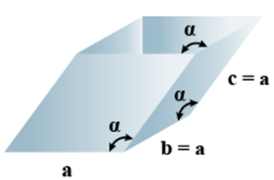• Bravais Lattices:
Primitive = 1
• Parameters of Unit Cell
(i) Intercepts: a = b= c
(ii) Crystal angle: α = γ = 90o, β≠ 90o
Example:  As, Sb, Bi, CaCO3

The table given below can be used to summarize types of lattice formation.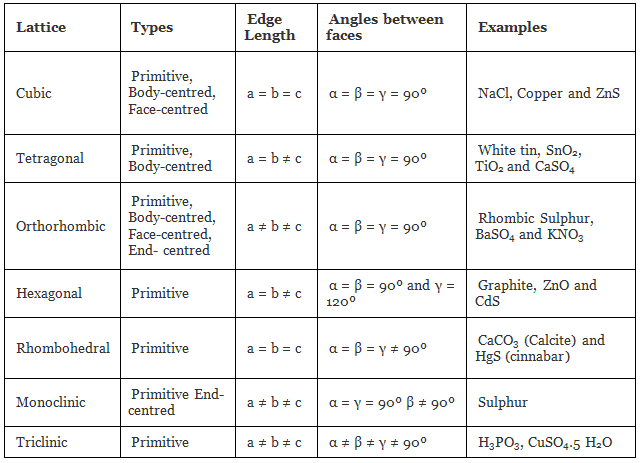Solved Examples

Q.1: Lithium borohydride crystallizes in an orthorhombic system with 4 molecules per unit cell. The unit cell dimensions are a = 6.8 Å,  b = 4.4 Å and C = 7.2 Å. If the molar mass is 21.76 g. Calculate the density of the crystal.
Solution:
Since, Density,Here z = 4, Av. No = 6.023 x 1023 &
Volume = V = a x b x c
= 6.8 x 108 x 4.4 x 108 x 7.2 x 108 cm
= 2.154 x 1022 cm3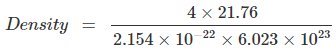= 0.6708 gm/cm3

Q.2. An element crystallizes into a structure which may be described by a cubic type of unit cell having one atom in each corner of the cube and two atoms on one of its face diagonals. If the volume of this unit cell is 24 x 10-24 cm3 and density of the element is 7.20 gm/cm3, calculate no. of atoms present in 200 gm of the element.
Ans: Number of atoms contributed in one unit cell = one atom from the eight corners + one atom from the two face diagonals = 1+1 = 2 atoms
Mass of one unit cell = volume × its density
= 24 × 10–24 cm3 × 7.2 gm cm3
= 172.8 × 10–24 gm
∴ 172.8 10–24 gm is the mass of one – unit cell i.e., 2 atoms
∴ 200 gm is the mass = 2 × 200 / 172.8 × 10–24 atoms = 2.3148 × 1024 atoms

Q.3. Calculate the void fraction for the structure formed by A and B atoms such that A form hexagonal closed packed structure and B occupies 2/3 of octahedral voids. Assuming that B atoms exactly fitting into octahedral voids in the HCP formed by A.
Ans: Total volume of A atom = 6 × 4 / 3 πrA3
Total volume of B atoms = 4 × 4/3 πrA3
=4 × 4/3 π(0.414rA)3
Since rB/rA as B is in octahedral void of A
Volume of HCP = 24√2rA3
Packing fraction = 6 × 4/3 πrA3 + 4 × 4/3 π (0.414rA)3 / 24√2rA3 = 0.7756
Void fraction = 1-0.7756 = 0.2244

Q.4: An element with molar mass 2.7×10-2kg mol-1 forms a cubic unit cell with edge length 405 pm. If its density is 2.7×103kg-3, what is the nature of the cubic unit cell?
Ans: d = 2.7×103kg-3
M = 2.7×10 -kg mol-1
a= 405 pm = 405 X 10-12
NA= 6.023 X 1023
Using the formula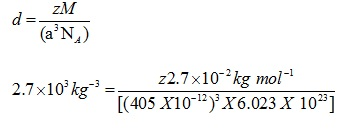Z=4
Unit cell is fcc unit cell.
Volume of 54 g of the element = 0.054/(2.7 x 103) = 2 X 10-6
Number of unit cell in this volume = volume of 554 g of element/volume of each unit cell = 2 x 10-6/(405 x 10-12)3 = 3.012 x 1022

Offer running on EduRev: Apply code STAYHOME200 to get INR 200 off on our premium plan EduRev Infinity!

## Chemistry for JEE

198 videos|450 docs|370 tests

,

,

,

,

,

,

,

,

,

,

,

,

,

,

,

,

,

,

,

,

,

;